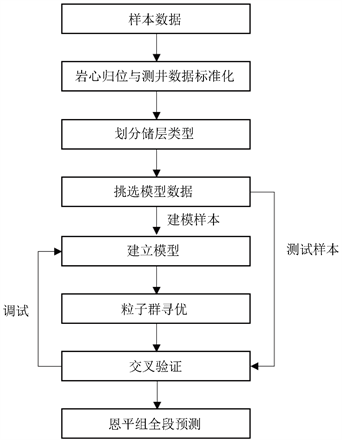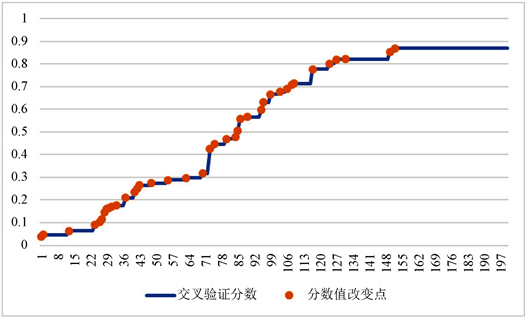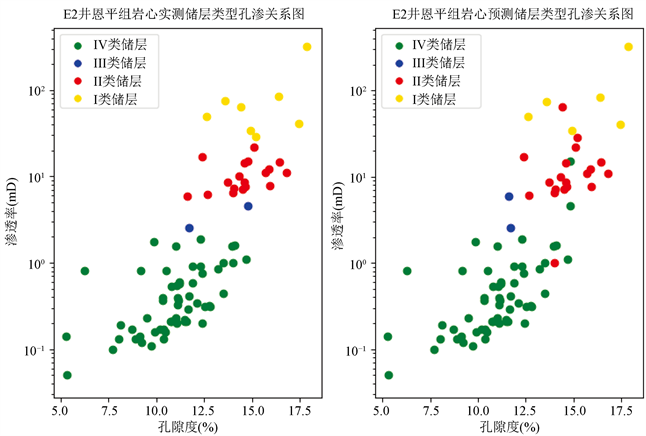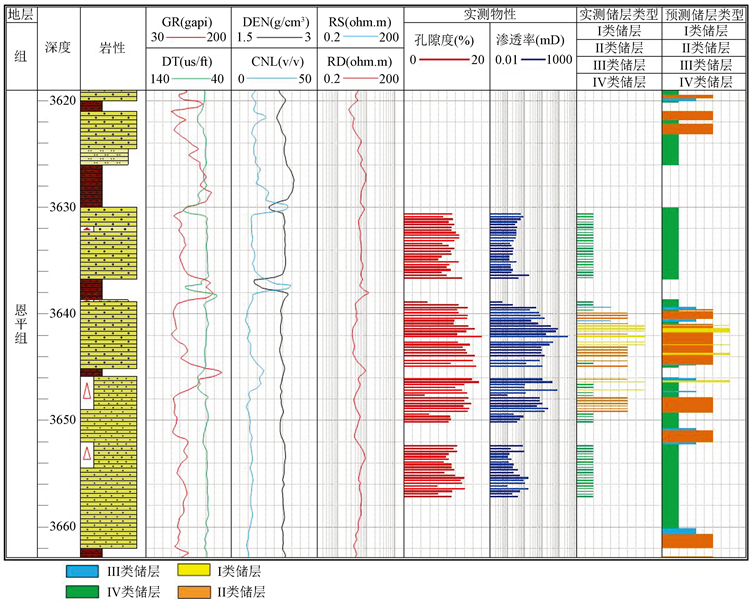# 粒子群寻优支持向量机在储层类型预测中的应用Application of Particle Swarm Optimization Support Vector Machine in Reservoir Prediction

• 全文下载: PDF(2050KB)    PP.18-26   DOI: 10.12677/AG.2020.101003
• 下载量: 91  浏览量: 121

In view of the problem that it is difficult to predict reservoirs in tight sandstone reservoirs of Enping formation in area of Zhu I depression, SVC is used to predict porosity of reservoirs by logging interpretation. The correctness of the model is improved by combining SVC with particle swarm optimization. The reservoir types of Enping formation are classified by typical well test and production test analysis. 80% of the samples of each type of reservoir are selected as modeling data, and random cross validation is carried out in each type of reservoir to get the cross-validation score of the model. Then the particle optimization method is used to improve the cross-validation score of the model to get the best prediction model. Then the prediction model is tested with the samples that are not involved in the modeling. From this model, the well log interpretation map can be made, that is, the location of the well section where the effective reservoir is located can be directly seen and the thickness of effective reservoir can also be calculated conveniently.

1. 引言

2. 原理分析

2.1. 支持向量分类原理

$\left\{{x}_{1},{y}_{1},{x}_{2},{y}_{2},\cdots ,{x}_{i},{y}_{i}\right\},\left(i=1,2,\cdots ,n\right)$ (1)

$f\left(x\right)=\left(w\cdot x\right)+b$ (2)

$\mathrm{min}\frac{1}{2}\left(w\cdot w\right)+C{\sum }_{i=1}^{n}\left({\xi }_{i}+{\xi }_{i}^{*}\right)$ (3)

$\text{s}.\text{t}.\left\{\begin{array}{c}{y}_{i}-\left(w\cdot {x}_{i}\right)-b\le \epsilon +{\xi }_{i}\\ \left(w\cdot {x}_{i}\right)+b-{y}_{i}\le \epsilon +{\xi }_{i}^{*}\\ \epsilon \ge 0,i=1,2,\cdots ,n\end{array}$ (4)

$\left\{\begin{array}{c}\mathrm{max}\phi \left(a\right)={\sum }_{i=1}^{n}\left({a}_{i}-{a}_{i}^{*}\right){y}_{i}-\frac{1}{2}{\sum }_{i,j=1}^{n}\left({a}_{i}-{a}_{i}^{*}\right)\left({a}_{j}-{a}_{j}^{*}\right)\left({x}_{i}\cdot {x}_{j}\right)\\ \text{s}.\text{t}.{\sum }_{i=1}^{n}\left({a}_{i}-{a}_{i}^{*}\right)=0\\ {a}_{i},{a}_{i}^{*}\ge 0\end{array}$ (5)

$f\left(x\right)={\sum }_{i=1}^{n}\left({a}_{i}-{a}_{i}^{*}\right)K\left({x}_{i},x\right)+b$ (6)

$K\left({x}_{i},x\right)=\mathrm{exp}\left\{-\frac{{\left({x}_{i}-x\right)}^{2}}{2{\sigma }^{2}}\right\}$ (7)

$f\left(x\right)={\sum }_{i=1}^{n}\left({a}_{i}-{a}_{i}^{*}\right)\mathrm{exp}\left[-\frac{{\left({x}_{i}-x\right)}^{2}}{2{\sigma }^{2}}\right]+b$ (8)

2.2. 粒子群寻优原理

${v}_{id}^{k+1}=\omega {V}_{id}^{k}+{c}_{1}{r}_{1}\left({P}_{id}^{k}-{X}_{id}^{k}\right)+{c}_{2}{r}_{2}\left({P}_{gd}^{k}-{X}_{id}^{k}\right)$ (9)

${X}_{id}^{k+1}={X}_{id}^{k}+{V}_{id}^{k+1}\text{\hspace{0.17em}}\left(i=1,2,\cdots ,m;d=1,2,\cdots ,D\right)$ (10)

3. 改进支持向量回归预测模型

3.1. 数据标准化

${y}_{ij}=\frac{{x}_{ij}-{x}_{i}}{{s}_{i}}$ (11)

3.2. 样本分类与选择

3.2.1. 储层下限分类Table 1. Reservoir classification and evaluation of Enping formation in Zhu I depression

3.2.2. 样本选取Table 2. Sample distribution of Enping formation in well E2

3.3. 模型建立Figure 1. Establishment process of Enping prediction model

3.4. 预测结果及分析Figure 2. Process chart of particle swarm optimizationFigure 3. Comparison of the relationship between the measured core and the predicted reservoir type porosity and permeability in Enping formation of well E2Table 3. Statistical table for prediction accuracy of reservoir type of measured samples in well E2Figure 4. Reservoir type prediction map of Enping formation in well E2

4. 结论

1) 粒子群寻优的收敛速度快，与传统网格搜索法相比，具有速度快、准确率高的特点 。将其运用到对支持向量机回归模型的参数c和 ${\delta }^{2}$ 的寻优中，可以得到相对误差较低，精确度较高的预测模型。

2) 根据储层下限对实测样本进行分类，可以在建立预测模型时确保建模样本的多样性。在检验模型时，对测试样本正确率进行分类统计，可以看出模型对于每类储层的预测精确度，对模型精确度不高的储层可以适量增加该类储层在建模样本中所占的比例。

3) 将模型运用于检验井恩平组全段砂岩的储层类型预测，可以较为准确的得到优质储层所在位置及优质储层的厚度。

  徐壮, 石万忠, 翟刚毅, 等. 涪陵地区页岩总孔隙度测井预测[J]. 石油学报, 2017, 38(5): 533-543.  胡作维, 李云. 基于偏最小二乘法评价低渗透砂岩储层质量[J]. 特种油气藏, 2013, 20(5): 36-39.  范雯. 逐步回归分析方法在储层参数预测中的应用[J]. 西安科技大学学报, 2014, 34(3): 350-355.  陈文浩, 王志章, 董少群, 等. 核岭回归方法解释致密砂岩储层孔隙度[J]. 测井技术, 2015, 39(6): 710-714.  吕晓光, 杜庆龙. 应用人工神经网络模型进行油层孔隙度, 渗透率预测[J]. 大庆石油地质与开发, 1996(3): 27-31.  Iqbal, M. (2005) Numerical Solutions of Linear Ill-Posed Problems. Integral Transforms & Special Functions, 16, 29-37.https://doi.org/10.1080/1065246042000271992  Phillips, D.L. (1962) A Technique for the Numerical Solution of Certain Integral Equations of the First Kind. Journal of the ACM, 9, 84-97.https://doi.org/10.1145/321105.321114  Cortes, C. and Vapnik, V. (1995) Support-Vector Networks. Machine Learning, 20, 273-297. https://doi.org/10.1007/BF00994018  乐友喜, 袁全社. 支持向量机方法在储层预测中的应用[J]. 石油物探, 2005, 44(4): 388-392.  滕新保, 张宏兵, 曹呈浩, 等. 一种新的砂泥岩孔隙度估计模型及其应用[J]. 河海大学学报: 自然科学版, 2015, 43(4): 346-350.  张向君, 张晔. 基于支持向量机的交互检验储层预测[J]. 石油物探, 2018, 57(4): 597-600.  李建军, 伦墨华. 基于支持向量机的石油勘探预测[J]. 科技通报, 2018(4): 79-83.  施和生, 雷永昌, 吴梦霜, 等. 珠一坳陷深层砂岩储层孔隙演化研究[J]. 地学前缘, 2008, 15(1): 169-175.  孟倩, 马小平, 周延. 改进的粒子群支持向量机预测瓦斯涌出量[J]. 矿业安全与环保, 2015(2): 1-5.  张燕君, 王会敏, 付兴虎, 等. 基于粒子群支持向量机的钢板损伤位置识别[J]. 中国激光, 2017(10): 197-203.  帅勇, 宋太亮, 王建平. 考虑全过程优化的支持向量机预测方法[J]. 系统工程与电子技术, 2017, 39(4): 931-940.  Xiong, W.L. and Xu, B.G. (2006) Study on Optimization of SVR Parameters Selection Based on PSO. Journal of System Simulation, 9, 2442-2445.  付超, 林年添, 张栋, 等. 多波地震深度学习的油气储层分布预测案例[J]. 地球物理学报, 2018, 61(1): 293-303.